What are they and Why?

Firstly what they are.

A Bézier curve is, in its simplest form, just a line from one point to another. It has a start point, and an end point.

However, it can be extended with what I call 'Helper Points'. These helper points act as guides to control the direction of the line when it starts and ends. So if you have a four-point Bézier Curve (start point, end point, and two helpers in the middle), then the curve will go like this: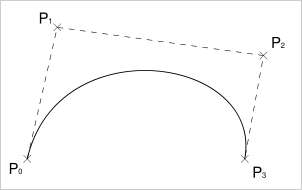Starts at p1 (Start point), facing p2 (first helper).
It goes towards p2, but long before it hits p2 it will start to curve off towards p3 (second helper).
Long before it reaches p3, it will start to curve again, this time towards p4 (end point).
It finally hits p4, and the line has finished.

That's a 4th-order Curve (otherwise known as a Cubic Curve).

The basic kinds of Bézier you can have are as follows:- 2nd Order - Linear - Just two points, this is a straight line. But it's crucial to know how straight lines work, because straight lines are the key to doing the Bézier Curve, as you'll see later.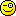- 3rd Order - Quadratic Curve - Three points. The line exits p1 aiming for p2. Before reaching p2, it changes its mind and steers towards p3. It hits p3, and the curve ends. See below: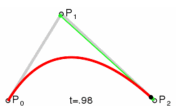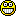- 4th Order - Cubic Curve - Four points, as explained above.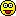- 5th Order and above - More than four points. These aren't very useful, since the more helper points you have, the more averaged out the curve becomes. It's better to combine lots of Cubic Curves than use one complex higher-order curve, so we won't consider how to do anything above the 4th Order.

Different curves obviously have different potential uses, but hopefully you can see from the basic explanations above what kind of things they can be used for.

Cubics are by far the best, because they let you set, not just the destination of an object, but also the angle that it will approach at. Imagine the uses for missiles! You can design a missile that will target the rear of a ship (where armour is weakest). As the enemy moves around, the missile will update its course in realtime to reach its target from the desired angle, like this: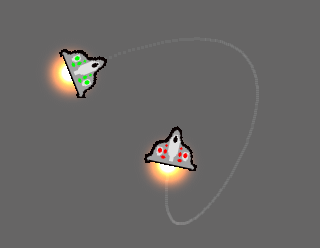You can also create these uber-mean-looking strider and tentacle creatures. And monsters with long curvy necks are easy to make too, using the helper points so that the snake-like body of the creature always enters the head from the back, and to firmly root its base to the floor so it bends realistically. The possibilities are endless.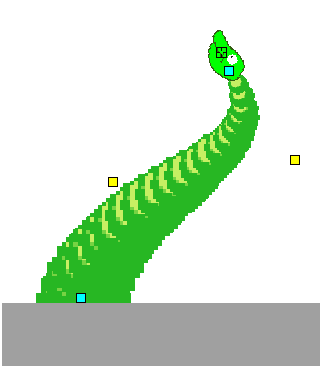You wanna add these things to your next platformer or sidescrolling sh'mup don't you?FORMULAE

What follows is our little explanation of the formulae for Béziers. Can I just point out right now that you do not need to be great at maths in order to understand these. Personally, I'm number dyslexic, but I managed to understand this even after reading much more complicated explanations than this will ever be. So seriously, you'll be fine, they're pretty logical.2nd Order - Basic Lines

This is the formula for linear curves (which are just straight lines actually):

p1 + (p2 - p1) * t

The meaning of the symbols is as follows:
p1 = Point 1's X or Y position (start point)
p2 = Point 2's X or Y position (end point)
t = Time. This is a decimal value between 0 and 1. At 0, you're at the start, and at 1, you're at the end. If you use the above formula on an object, then gradually increasing t will cause the object to move along the curve.

What it's doing is pretty simple. It finds the distance between the two points (p2 - p1), and it gets just a fraction of that distance, however much is needed by t. So if t is 0.5, it'll only return half the total distance.
It then adds that to the position of the first point, and you're done.

That'll plot the coordinates for your line. Just keep increasing t, and you'll get a neat little line.

Just a little warning now; for Béziers that have more than two points, t isn't regular. You'll find this when you test it. If you increase t by 0.1 every frame, and you draw a dot along the curve every frame, you'll find that the dots are NOT equally spaced. That's an inherent problem with Bézier Curves, and it's unavoidable because of the way they work.

Anyway, if you wanted to apply the above formula in MMF, you'd do something like this:
Create three objects (a start and end point, and a follower).
Give the follower an Alterable Value - we'll call it t.

Set X of follower to:
x(Start) + (x(End) - x(Start)) * t(Follower)

Set Y of follower to:
y(Start) + (y(End) - y(Start)) * t(Follower)

Hopefully, that's fairly simple.It's just p1 + (p2 - p1) * t

You've probably already made one of these before, although I doubt they called it a quadratic curve, LOL!
At primary/elementary school, sometimes the teachers get kids to draw a right angled triangle, and connect it with lines, like this: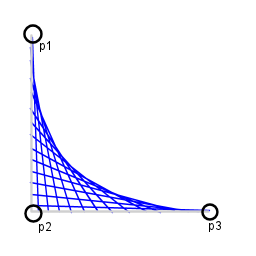See the curve that's formed? Well that's a 3rd Order Bézier Curve in all its glory!Say hello to the curve!!

The key to drawing it in MMF is very similar to the above... it just needs more lines! Remember how I said that lines are the key to Béziers? Well I hope you remembered that basic formula above, cos you're gonna need it!!p1 + (p2 - p1) * t = q1;

p2 + (p3 - p2) * t = q2;

q1 + (q2 - q1) * t

What we're doing here is using TWO lines. I've called them q1 and q2. As t gradually increases, it keeps giving us a coordinate for q1 and q2 (as we move further and further along those lines).

Instead of putting an object along those lines, as we did with the straight line, we use those coordinates to plot a THIRD line between them. This is the line we're gonna actually draw.

Since the values of q1 and q2 are constantly changing (as t changes), so is the line drawn between the two. We move along this third line using t as well, and the result is a Quadratic Bézier Curve.This nifty animation is hosted by Wikipedia, and I think it explains what's going on quite neatly:-- NOTE: Using it in MMF --

We need to break away just for a second here, cos there's a teensy weensy problem with the above. The problem is that MMF won't let you define variables in the expression editor. So our formula would instead become:

(p1 + (p2 - p1) * t) + ((p2 + (p3 - p2) * t) - (p1 + (p2 - p1) * t)) * t

...and that's pretty ugly isn't it.

The problem with Bézier curves is that even though they are, in principle, fairly simple, they can end up looking like a very very ugly formula if you can't use variables to cut them down (like we did when I was explaining them to you). And it gets worse when there are four, five or more points to deal with!

To get around it, I would write the expression out like this first (you may prefer to do it in notepad, so you can use Find and Replace to automate this process):

x(p 1) + (x(p 2) - x(p 1)) * t(Follower) = q1

x(p 2) + (x(p 3) - x(p 2)) * t(Follower) = q2

q1 + (q2 - q1) * t(Follower)

-
Then, in a stroke of genius, I would select the q1 code (NOT including = q1), find where it says 'q1' in the final formula, surround it with ( brackets ) first, and then paste the code over the top. And the same with q2. The result?

(x(p 1) + (x(p 2) - x(p 1)) * t(Follower)) + ((x(p 2) + (x(p 3) - x(p 2)) * t(Follower))

- (x(p 1) + (x(p 2) - x(p 1)) * t(Follower))) * t(Follower)

-
Looks complicated, aye? But the important thing is, it wasn't complicated when I wrote it. The hard part was done when it was a nice simple little formula. Now it looks worse than porridge, but at least it's a porridge that only the MMF interpretter has to deal with. You may want to keep a record of the original, simplified version in Notepad. In case you bodged it and need to change something.

Alternatively, you could use Alterable Values to hold the formulae for q1 and q2. But that's just a waste of some perfectly good alterable value slots in my opinion.

4th Order - Cubic Curves

Here it is, the Daddy, and the most compelling reason for anyone to bother reading this article. The Cubic Bézier Curve is upon us!!

It's a little more complicated, so we... add more lines!!p1 + (p2 - p1) * t = q1
p2 + (p3 - p2) * t = q2
p3 + (p4 - p3) * t = q3

q1 + (q2 - q1) * t = r1
q2 + (q3 - q2) * t = r2

r1 + (r2 - r1) * t

(at this point, I'll accept that using Alterable Values could be a good idea!!)

It's literally just an extension of the 3rd-Order Curve. There are more lines, but they're still just lines.

We have four points, which connect into 3 lines as shown below. I've labelled the lines q1, q2 and q3.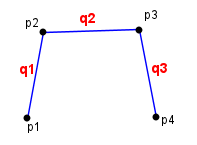These three lines are gonna become 3 sets of coordinates as we travel along them using t. Since we now have 3 sets of coordinates, we can treat them just like a Quadratic (3-point) Curve.

The final result is a Bézier Curve, YAY!Once again, here's the Wikipedia animation:If you don't care about learning and you just wanna use 'em...

Then okay, I won't stop you. You don't *have* to learn how Bézier curves work in order to use them, you can just copy and paste a formula. So if you fall into this category, and you don't appreciate wisdom, and you have no self respect, and you were raised by alien rodents, then here you go:

--- To Use:
Create an object for each point in the curve you want. Name each point like this:

p # where # is the number of the point. So if you're making a 4th-Order Curve (Cubic), you'll have some small active objects called 'p 1', 'p 2', 'p 3' and 'p 4'.

Also, create an object called 'F'. This will be your Follower object, and you can rename him after you've pasted the formula.
Give that object an alterable value called t.

NOTE: These may take some time to paste, as MMF will insist on colour-coding everything.